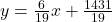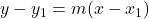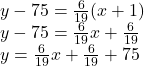## What is the point-slope form of the equation for the line with a slope of 6/19 that passes through the point (−1,75)?

Question

What is the point-slope form of the equation for the line with a slope of 6/19 that passes through the point (−1,75)?

in progress 0
1 month 2021-08-12T13:20:02+00:00 1 Answers 0 views 0

##Step-by-step explanation:

To find an equation of a line in point slope form when given the slope and a point we use the formulawhere

m is the slope

( x1 , y1) is the point

From the question the point is (−1,75) and slope 6/19 isWe have the final answer asHope this helps you